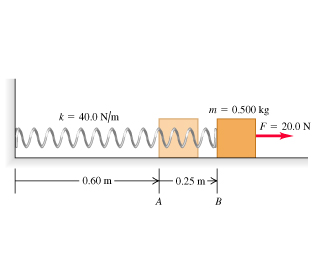# Problem: A 0.500-kg block, attached to a spring with length 0.60 m and force constant 40.0 N/m, is at rest with the back of the block at point exttip{A}{A} on a frictionless, horizontal air table (the figure ). The mass of the spring is negligible. You move the block to the right along the surface by pulling with a constant 20.0-N horizontal force.What is the blocks speed when the back of the block reaches point exttip{B}{B}, which is 0.25 m to the right of point exttip{A}{A}?When the back of the block reaches point exttip{B}{B}, you let go of the block. In the subsequent motion, how close does the block get to the wall where the left end of the spring is attached?

###### FREE Expert Solution
80% (488 ratings)
###### Problem Details

A 0.500-kg block, attached to a spring with length 0.60 m and force constant 40.0 N/m, is at rest with the back of the block at point on a frictionless, horizontal air table (the figure). The mass of the spring is negligible. You move the block to the right along the surface by pulling with a constant 20.0-N horizontal force.

What is the blocks speed when the back of the block reaches point , which is 0.25 m to the right of point ?

When the back of the block reaches point , you let go of the block. In the subsequent motion, how close does the block get to the wall where the left end of the spring is attached?

Frequently Asked Questions

What scientific concept do you need to know in order to solve this problem?

Our tutors have indicated that to solve this problem you will need to apply the Springs & Elastic Potential Energy concept. You can view video lessons to learn Springs & Elastic Potential Energy. Or if you need more Springs & Elastic Potential Energy practice, you can also practice Springs & Elastic Potential Energy practice problems.